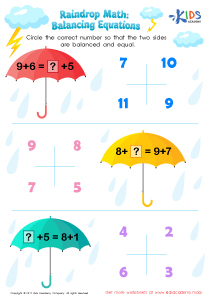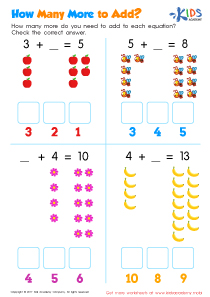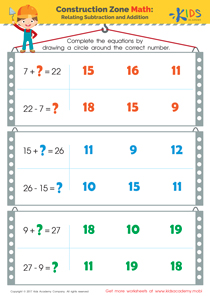• ### Activity 1 / Raindrop Math PrintableBalancing equations is important to show kids that multiple equations can result in the same answer. Use this balancing addition equations worksheet to encourage your child to think deeper about equations to understand math inside and out!
• Form a foundation for early algebraic thinking
• Gain a deeper sense of numeracy and understanding of equations
Kids learn much more than merely solving math problems in today’s classroom. Get your child on the fast track to higher level math, using this balancing equations worksheet!

• ### Activity 2 / How Many More to Add WorksheetSharpen your child’s addition and subtraction skills with the pdf worksheet. How many more apples, bees, flowers and bananas do you need to arrive at the figure provided? The possible answers to the unfinished math are provided at the bottom of the boxes. All you need to do with your child is do some quick addition and subtraction magic, and you can have some fun learning maths.

• ### Activity 3 / Related Addition and Subtraction Facts WorksheetRelating addition with subtraction helps builds your child’s sense of numeracy as he or she will soon understand how numbers truly work.
Offer your child related addition and subtraction facts worksheets like this one to help your child gain a deeper sense of numbers in a quick and enjoyable way!

?